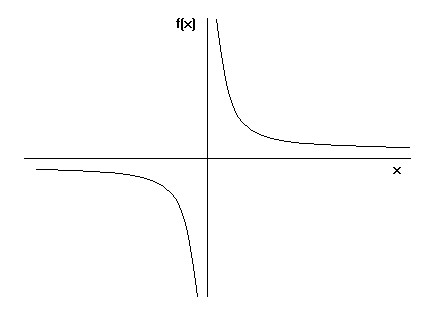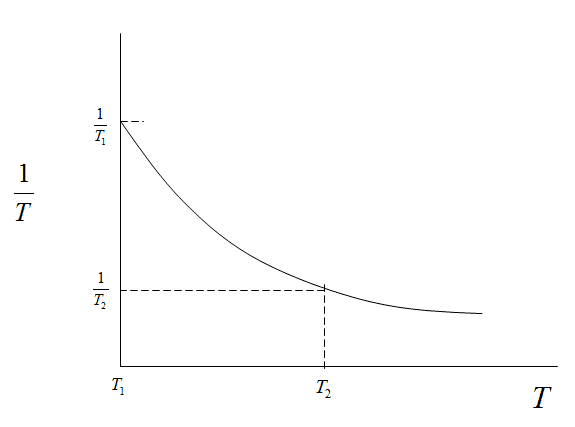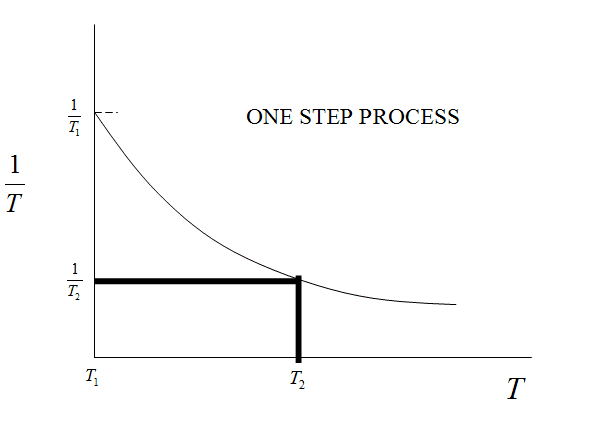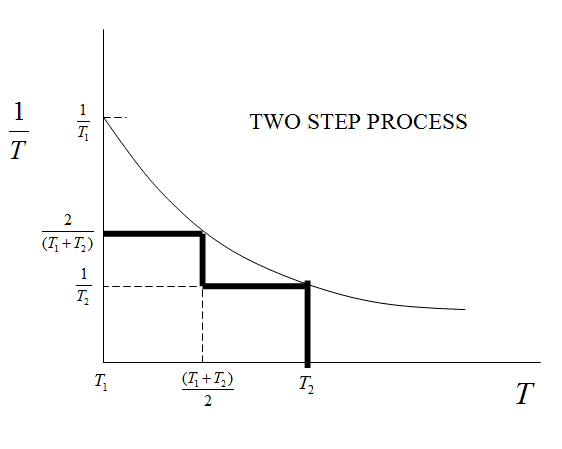# Heat transfer processes and reversibility

misko
I don't understand why heat transfer from hot reservoir to the system is considered reversible in case when
Treservoir = Tsystem + dT

but it's considered irreversible in this case:
Treservoir = Tsystem + ΔT

Where dT is infinitesimal difference while ΔT is finite difference in temperature between reservoir and the system.

In both cases some heat is transferred from the reservoir to the system, so it should be irreversible in both cases. What understanding am I missing here?

## Answers and Replies

Homework Helper
Gold Member
dT is infinitesimal

mfig
I don't understand why heat transfer from hot reservoir to the system is considered reversible in case when
Treservoir = Tsystem + dT

but it's considered irreversible in this case:
Treservoir = Tsystem + ΔT

This is because when only a differential temperature difference exists, the heat transfer is so small and takes so long to accomplish that time is effectively eliminated. This allows us to say there is "no disequilibrium" anywhere (or that the irreversibilities are infinitesimal everywhere) in the system between commencement of heat transfer and final thermal equilibrium. It is a quasi-static process. When the temperature difference is finite, on the other hand, we can no longer claim each state is an equilibrium state because of larger gradients that accompany actual heat transfer.

misko
I agree with you that infinitesimal dT is the key to have the reversible process, I read that in several places. But I don't understand why is that.

Let's say we have some system in thermal equilibrium with temperature T and let's also have two reservoirs with temperatures T and T+dT.
If we do following steps:
1) put the system in contact with reservoir T+dT and wait for system to increase it's temperature to T+dT
2) put the system in contact with reservoir with temperature T, and wait system to get back to original temperature T.
Then we have successfully returned the system to the initial state right? BUT, the surroundings is not returned to the initial state because we have irreversibly transferred some heat dQ between these two reservoirs. Since this change is left in the surroundings doesn't this means the process is actually irreversible?
Also if we calculate entropy change for the universe after this process we will have
ΔSsystem=0
ΔSsurroundings=-dQ/T+dT + dQ/T >0

What am I missing in this reasoning?

mfig
BUT, the surroundings is not returned to the initial state because we have irreversibly transferred some heat dQ between these two reservoirs. Since this change is left in the surroundings doesn't this means the process is actually irreversible?

No, we have not. By only holding the temperature difference to dT we have reversibly transferred heat. A reversible process is, by definition, one for which both the system and the environment can be returned to the initial state. Obviously, reversible processes are only hypothetical.

misko
I agree with you about the definition of reversible process and also that they are just theoretical idealization. I know that no process in nature is completely reversible, that's why I am speaking theoretically.

My question is this: has surrounding returned to the initial state after we performed these steps above?
If yes then we have reversible process. But in my head it seems like it did not. Obviously we have some heat transfer between reservoirs after the cycle. This heat came from the hotter reservoir to the colder and it stays that way in the surrounding. This heat can't go back to the colder reservoir spontaneously so it seems like this is irreversible change now. In my simple mind I don't understand why is this reversible process only because the heat transfer is infinitesimally small, it only means that the whole cycle was quasi-static.
So... probably I am missing something in this logic but still not getting it :(

Mentor
Suppose you have a body of mass M and heat capacity C at T1 and an infinite reservoir at T2>T1. You put them into thermal contact and allow them to equilibrate. The amount of heat transferred is ##Q=MC(T_2-T_1)##. The entropy change for the infinite reservoir is $$\Delta S_{res}=-MC\frac{(T_2-T_1)}{T_2}$$ and the entropy change for the body is $$\Delta S_{body}=MC\ln(T_2/T_1)$$So, the total change in entropy for the universe is $$\Delta S =MC\ln(T_2/T_1)-MC\frac{(T_2-T_1)}{T_2}>0$$OK so far?

Now suppose you have the same body at T1, but now you have two infinite reservoirs, one at (T1+T2)/2 and the other at T2. You bring about the same change in the system, but now using two separate steps in the following sequence:

Step 1. Put the body at temperature T1 into contact with the reservoir at (T1+T2)/2, and let them equilibrate
Step 2. Put the body at temperature (T1+T2)/2 into contact with the reservoir at T2, and let them equilibrate

What is the amount of heat transferred in each of these steps? What is the change in entropy of the body in each of these steps? What is the change in entropy of the infinite reservoirs in each of these steps? What is the total change in entropy of the universe in each of these steps? What is the change in entropy of the universe over the entire process? How does the change in entropy of the universe compare with the case in which the process was carried out in only one step?

Please bear with me. I know that this may not seem to be going anywhere to you, but please have faith, there is a method to my madness.

Chet

misko
Hello Chet,

I did the calculations (hopefully correctly) so here are my results:

Step 1:
$$Q=MC(T_2-T_1)/2$$
$$\Delta S_{body}=MC\ln(\frac{T_1+T_2}{2T_1})$$
$$\Delta S_{res}=-MC\frac{(T_2-T_1)}{T_1+T_2}$$

Step 2:
$$Q=MC(T_2-T_1)/2$$
$$\Delta S_{body}=MC\ln(\frac{2T_2}{T_1+T_2})$$
$$\Delta S_{res}=-MC\frac{(T_2-T_1)}{2T_2}$$

Total entropy of the universe for step 1 and step 2:
$$\Delta S =MC\ln(T_2/T_1)-MC\frac{(T_2-T_1)}{T_2}\frac{(3T_2+T_1)}{2(T_1+T_2)}$$

So it seems that the total entropy in this case differs by the last member in the equation: ##\frac{(3T_2+T_1)}{2(T_1+T_2)}## which is > 1.
This then makes the total entropy in this 2-step process smaller than the total entropy we got the first case when we had process with only one step.

Did I get this right?

Mentor
Hello Chet,

I did the calculations (hopefully correctly) so here are my results:

Step 1:
$$Q=MC(T_2-T_1)/2$$
$$\Delta S_{body}=MC\ln(\frac{T_1+T_2}{2T_1})$$
$$\Delta S_{res}=-MC\frac{(T_2-T_1)}{T_1+T_2}$$

Step 2:
$$Q=MC(T_2-T_1)/2$$
$$\Delta S_{body}=MC\ln(\frac{2T_2}{T_1+T_2})$$
$$\Delta S_{res}=-MC\frac{(T_2-T_1)}{2T_2}$$

Total entropy of the universe for step 1 and step 2:
$$\Delta S =MC\ln(T_2/T_1)-MC\frac{(T_2-T_1)}{T_2}\frac{(3T_2+T_1)}{2(T_1+T_2)}$$
Very nicely done. I would like to write the result in a little different mathematical form:

$$\Delta S =MC\ln(T_2/T_1)-MC\frac{(T_2-T_1)}{T_2}\left[1+\frac{(T_2-T_1)}{2(T_1+T_2)}\right]$$This illustrates more explicitly that the entropy change for the reservoirs becomes more negative.
So it seems that the total entropy in this case differs by the last member in the equation: ##\frac{(3T_2+T_1)}{2(T_1+T_2)}## which is > 1.
This then makes the total entropy in this 2-step process smaller than the total entropy we got the first case when we had process with only one step.

Did I get this right?
Yes. good job. So the entropy change of the universe for the two step process is less than the one step process. In this sense, the 2-step process was "less irreversible."

What do you think would happen if we went to 4 steps? What do you think would happen if we went to 100 steps? Would the total entropy change of the reservoirs continue to become more negative (and the entropy change of the universe become less positive) as we increased the number of steps? Would there be a limit to how negative the combined entropy changes of the sequence of reservoirs would get as we increased the number of steps? Would there be a limit to how small the entropy change of the universe would get as we increased the number of reservoirs?

I'd like you to try something: Make a rough sketch showing 1/T plotted as a function of T, showing the region on the T axis between ##T_1## and ##T_2##. See if you can illustrate on the graph the calculation we have done to get the entropy change of the reservoirs in the 1-step process and the 2-step process. If you have trouble with this, I will help after you respond back.

Chet

misko
Hello Chet,

I will try to answer your questions by using intuition and the result of the calculations I got.

What do you think would happen if we went to 4 steps? What do you think would happen if we went to 100 steps? Would the total entropy change of the reservoirs continue to become more negative (and the entropy change of the universe become less positive) as we increased the number of steps?

I think that entropy of the reservoirs would get more negative and total entropy of the universe would get less positive as we increase number of steps.

Would there be a limit to how negative the combined entropy changes of the sequence of reservoirs would get as we increased the number of steps? Would there be a limit to how small the entropy change of the universe would get as we increased the number of reservoirs?

So I suppose that in the limiting case when number of steps goes to infinity we would get total entropy of the universe to be zero. In order for this to happen I think that positive entropy change of the system should cancel out with the negative entropy change of the reservoirs.
This means that in the following equation:
$$\Delta S =MC\ln(T_2/T_1)-MC\frac{(T_2-T_1)}{T_2}\left[1+\frac{(T_2-T_1)}{2(T_1+T_2)}\right]$$
we will have this term ##\frac{(T_2-T_1)}{T_2}\left[1+\frac{(T_2-T_1)}{2(T_1+T_2)}\right]## somehow becoming equal to the ##\ln(T_2/T_1)## when number of steps goes to infinity. Though I am not sure how exactly the generalized term for ##\Delta S_{reservoirs}## should look like if we have ##n## steps.

I'd like you to try something: Make a rough sketch showing 1/T plotted as a function of T, showing the region on the T axis between T1T1T_1 and T2T2T_2. See if you can illustrate on the graph the calculation we have done to get the entropy change of the reservoirs in the 1-step process and the 2-step process. If you have trouble with this, I will help after you respond back.

I am not sure how to do this. I mean, plotting the 1/T as a function of T is just plotting the graph f(x) = 1/x which looks like this:but I am not sure how to put on this graph the entropy calculations we did.

Mentor
Hello Chet,

I will try to answer your questions by using intuition and the result of the calculations I got.

I think that entropy of the reservoirs would get more negative and total entropy of the universe would get less positive as we increase number of steps.

So I suppose that in the limiting case when number of steps goes to infinity we would get total entropy of the universe to be zero. In order for this to happen I think that positive entropy change of the system should cancel out with the negative entropy change of the reservoirs.
This means that in the following equation:
$$\Delta S =MC\ln(T_2/T_1)-MC\frac{(T_2-T_1)}{T_2}\left[1+\frac{(T_2-T_1)}{2(T_1+T_2)}\right]$$
we will have this term ##\frac{(T_2-T_1)}{T_2}\left[1+\frac{(T_2-T_1)}{2(T_1+T_2)}\right]## somehow becoming equal to the ##\ln(T_2/T_1)## when number of steps goes to infinity. Though I am not sure how exactly the generalized term for ##\Delta S_{reservoirs}## should look like if we have ##n## steps.
The "reservoirs contribution" in the equation would change as you added more reservoirs, and continued to reduce the amount of heat transferred in each individual step. For 4 steps, the "reservoirs contribution" would be the sum of 4 terms. I will now show you graphically what is happening when you add more reservoirs.

The figure below shows a schematic plot of 1/T vs T. Shown on the T axis are the values of ##T_1## and ##T_2##. Also shown on the plot are the value of 1/T at ##T_1## and ##T_2##, namely, ##1/T_1## and ##1/T_2##.Now let's see how this relates to the 1 step process and the 2 step process. Let's first consider the graph for the 1 step process. This is shown in the figure below:The area of the rectangle in the figure is ##\frac{T_2-T_1}{T_2}##. Compare that with the temperature term for the entropy change of the reservoir in my post #7 for the 1 step process. The two are identical. So the area under the thick black curve in the figure is identical to the temperature term in the equation for the entropy change of the reservoir in the 1 step process. Next, let's consider the graph for the 2 step process. This is shown in the figure below.The area under the thick black curve in this figure is equal to ##\frac{T_2-T_1}{2}\left(\frac{1}{T_2}+\frac{2}{(T_1+T_2)}\right)##. Compare this with the temperature terms in your equations for the entropy changes of the two reservoirs in post #8. The two are identical. So the area under the thick black curve in the figure is identical to the temperature term in the equation for the entropy change of the reservoirs in the 2 step process.

Can you see where the area under the thick black curve in the figure for the 2 step process is a better approximation to the area under the curve of 1/T vs T (i.e., the integral of dT/T) than the area under the thick black curve in the figure for the 1 step process? Can you visualize what would happen graphically as we added more reservoirs and steps to the process?

misko
Hello Chet,
I understand the graphs you provided thanks. I see where it is going: as we add more and more reservoirs in the process the area consisting of rectangles under the curve will get better and better at approximating the are under the cure of 1/T vs T.
So in the limiting case (where number of reservoirs goes to infinity) this would then become what we call a quasi-static process and we will have matching between these areas.
I suppose that total entropy change in this limiting case should then be zero?
Also I wanted to ask why did you use 1/T vs T graph, what is the physical meaning behind using 1/T?

Thanks for the effort of explaining this to me, I really appreciate it!

Mentor
Hello Chet,
I understand the graphs you provided thanks. I see where it is going: as we add more and more reservoirs in the process the area consisting of rectangles under the curve will get better and better at approximating the are under the cure of 1/T vs T.
So in the limiting case (where number of reservoirs goes to infinity) this would then become what we call a quasi-static process and we will have matching between these areas.
I suppose that total entropy change in this limiting case should then be zero?
Sure.
Also I wanted to ask why did you use 1/T vs T graph, what is the physical meaning behind using 1/T?
How do you go about determining the entropy change for the body in raising its temperature from T1 to T2 (no just the equation I wrote, but the rationale behind its derivation)?